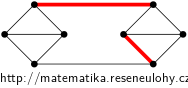## Two unmatchable edges

Construct a cubic graph without bridges that contains two non-adjacent edges that do not together belong to any common matching.

• #### Hint

Explore matching of a small complete graph and also possible operations with this graph.

• #### Solution

The complete graph $$K_4$$ has a unique partition into perfect matchings. Take two copes of $$K_4$$, in each choose an edge, delete these two and then add edges between vertices of degree two to make this graph connected.

The newly added edges can be extended to a unique matching, so it is easy to pick one of them and a non-matchable edge from the rest, see the picture.